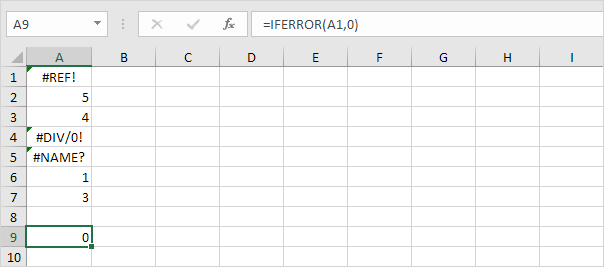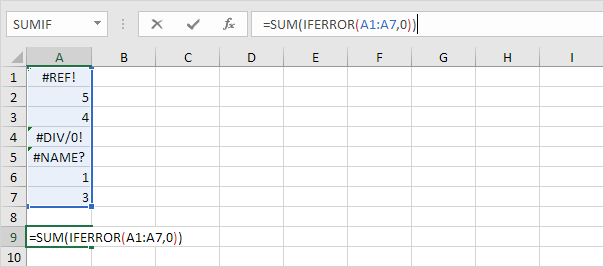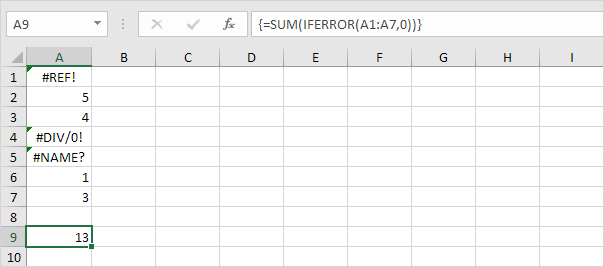# Sum Range with Errors

This example shows you how to create an array formula that sums a range with errors. You can also use the AGGREGATE function to sum a range with errors.

1. We use the IFERROR function to check for an error.Explanation: the IFERROR function returns 0, if an error is found. If not, it returns the value of the cell.

2. To sum the range with errors (don't be overwhelmed), we add the SUM function and replace A1 with A1:A7.3. Finish by pressing CTRL + SHIFT + ENTER.Note: the formula bar indicates that this is an array formula by enclosing it in curly braces {}. Do not type these yourself. They will disappear when you edit the formula. In Excel 365 or Excel 2021, finish by simply pressing Enter. You won't see curly braces.

Explanation: the range (array constant) created by the IFERROR function is stored in Excel's memory, not in a range. The array constant looks as follows:

{0;5;4;0;0;1;3}

This array constant is used as an argument for the SUM function, giving a result of 13.

Go to Next Chapter: Sort